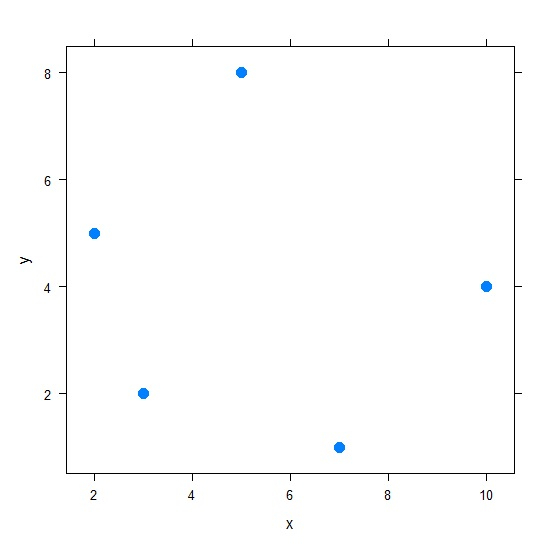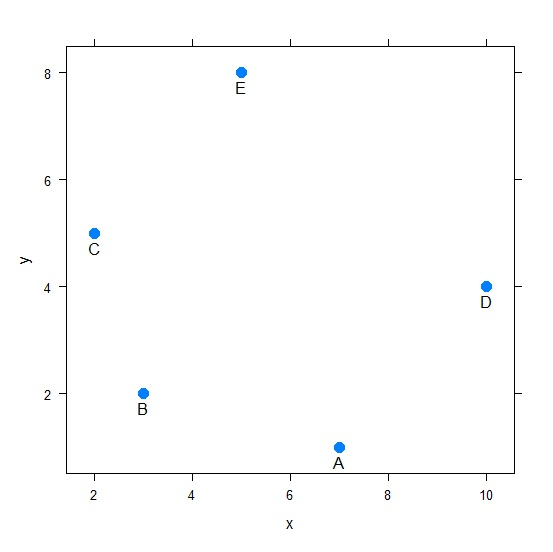# How to label points in scatterplot created by using xyplot in R?

To label points in scatterplot created by using xyplot, we can follow the below steps −

• First of all, creating two numerical and one categorical vector.
• Creating the scatterplot with points labelled using categorical vector by panel.xyplot and ltext function.

## Creating the vectors

Create three vectors, one categorical and two numerical −

Live Demo

x<-sample(1:10,5)
y<-sample(1:10,5)
Dots<-c("A","B","C","D","E")
x
y
Dots

On executing, the above script generates the below output(this output will vary on your system due to randomization) −

 7 3 2 10 5
 1 2 5 4 8
 "A" "B" "C" "D" "E"

Use xyplot function to create the scatterplot between x and y −

library(lattice)
xyplot(y~x,data=data.frame(x,y),pch=20,cex=2)

### Output## Creating the scatterplot with labels

Use panel.xyplot and ltext to create the scatterplot with labels −

x<-sample(1:10,5)
y<-sample(1:10,5)
Dots<-c("A","B","C","D","E")
xyplot(y~x,data=data.frame(x,y),pch=20,cex=2,panel=function(x,y,...){
+ panel.xyplot(x,y,...);
+ ltext(x=x,y=y,labels=Dots,pos=1)})

### Output